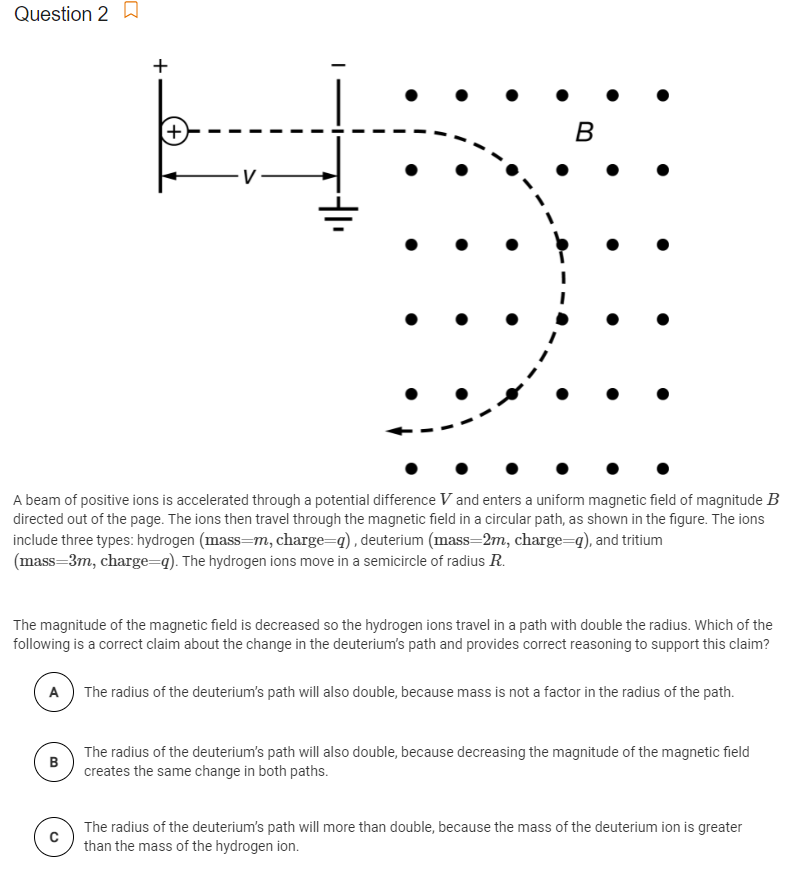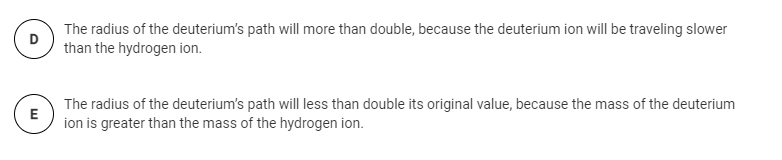# Question Solved1 AnswerQuestion 2 a + + B A beam of positive ions is accelerated through a potential difference V and enters a uniform magnetic field of magnitude B directed out of the page. The ions then travel through the magnetic field in a circular path, as shown in the figure. The ions include three types: hydrogen (mass=m, charge=9), deuterium (mass=2m, charge=q), and tritium (mass=3m, charge=q). The hydrogen ions move in a semicircle of radius R. The magnitude of the magnetic field is decreased so the hydrogen ions travel in a path with double the radius. Which of the following is a correct claim about the change in the deuterium's path and provides correct reasoning to support this claim? A The radius of the deuterium's path will also double, because mass is not a factor in the radius of the path. B The radius of the deuterium's path will also double, because decreasing the magnitude of the magnetic field creates the same change in both paths. С The radius of the deuterium's path will more than double, because the mass of the deuterium ion is greater than the mass of the hydrogen ion. D The radius of the deuterium's path will more than double, because the deuterium ion will be traveling slower than the hydrogen ion. E The radius of the deuterium's path will less than double its original value, because the mass of the deuterium ion is greater than the mass of the hydrogen ion.Transcribed Image Text: Question 2 a + + B A beam of positive ions is accelerated through a potential difference V and enters a uniform magnetic field of magnitude B directed out of the page. The ions then travel through the magnetic field in a circular path, as shown in the figure. The ions include three types: hydrogen (mass=m, charge=9), deuterium (mass=2m, charge=q), and tritium (mass=3m, charge=q). The hydrogen ions move in a semicircle of radius R. The magnitude of the magnetic field is decreased so the hydrogen ions travel in a path with double the radius. Which of the following is a correct claim about the change in the deuterium's path and provides correct reasoning to support this claim? A The radius of the deuterium's path will also double, because mass is not a factor in the radius of the path. B The radius of the deuterium's path will also double, because decreasing the magnitude of the magnetic field creates the same change in both paths. С The radius of the deuterium's path will more than double, because the mass of the deuterium ion is greater than the mass of the hydrogen ion. D The radius of the deuterium's path will more than double, because the deuterium ion will be traveling slower than the hydrogen ion. E The radius of the deuterium's path will less than double its original value, because the mass of the deuterium ion is greater than the mass of the hydrogen ion.
More
Transcribed Image Text: Question 2 a + + B A beam of positive ions is accelerated through a potential difference V and enters a uniform magnetic field of magnitude B directed out of the page. The ions then travel through the magnetic field in a circular path, as shown in the figure. The ions include three types: hydrogen (mass=m, charge=9), deuterium (mass=2m, charge=q), and tritium (mass=3m, charge=q). The hydrogen ions move in a semicircle of radius R. The magnitude of the magnetic field is decreased so the hydrogen ions travel in a path with double the radius. Which of the following is a correct claim about the change in the deuterium's path and provides correct reasoning to support this claim? A The radius of the deuterium's path will also double, because mass is not a factor in the radius of the path. B The radius of the deuterium's path will also double, because decreasing the magnitude of the magnetic field creates the same change in both paths. С The radius of the deuterium's path will more than double, because the mass of the deuterium ion is greater than the mass of the hydrogen ion. D The radius of the deuterium's path will more than double, because the deuterium ion will be traveling slower than the hydrogen ion. E The radius of the deuterium's path will less than double its original value, because the mass of the deuterium ion is greater than the mass of the hydrogen ion.